## General Question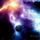# How much soil is there in a hole measuring one metre by one metre by one metre?

Asked by wingsoffire (31) July 4th, 2007
Observing members: 0Composing members: 0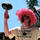Uh, one cubic meter. Is this a trick question?

segdeha (1707)“Great Answer” (0) Flag as…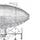I think wingsoffire intended it thus: There isn't any soil in a hole.

However, There is if it's a hole in the concrete that's filled with soil.

Or a hole in the sand filled with soil (so you can plant things that don't grow in sand).

There *was* one cubic meter of soil in the hole, which is now located somewhere else, and entropically wants to fill the hole back in.

bpeoples (2546)“Great Answer” (3) Flag as…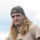I just want to know how you dug a perfectly square hole. Every hole that I've ever dug is round and has a roundish bottom. Props on making a square hole :D

Perchik (4982)“Great Answer” (2) Flag as…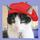Why? Plants love BIG and DEEP; they don't care about right angles. For construction, you need props or supports.

gailcalled (54570)“Great Answer” (0) Flag as…Sheets of metal or plywood, if used for a short time.

gailcalled (54570)“Great Answer” (0) Flag as…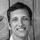For a hemispherical hole with a radius of a half meter (i.e., one meter across and a half meter deep), the answer is ((4/3)π(.5³))/2 = 0.261799388 cubic meters. For a cylindrical hole with a radius and depth of a half meter, the answer is π(.5²)/2 = 0.392699082 cubic meters. Assuming that a real one-by-one-by-one-meter hole resembles a cylinder stacked atop a hemisphere (both with half-meter radii), the volume of that hole would be the sum of the two previous calculations or 0.65449847 cubic meters.

sferik (6099)“Great Answer” (0) Flag as…sferik (6099)“Great Answer” (0) Flag as…I just noticed that you asked "how much" soil, so you might want the answer in weight instead of volume (I believe soil is normally sold by weight). Assuming an average dry, packed soil density of ρ = 1,500 kilograms per cubic meter, and taking my volume estimation from above there would be 982 kilograms of soil in the hole (or 1,500 kilograms if the hole is a cube).

sferik (6099)“Great Answer” (2) Flag as…or